# How to Convert Google Sheets to Excel Automatically

Learn to automate conversion for seamless data transfer.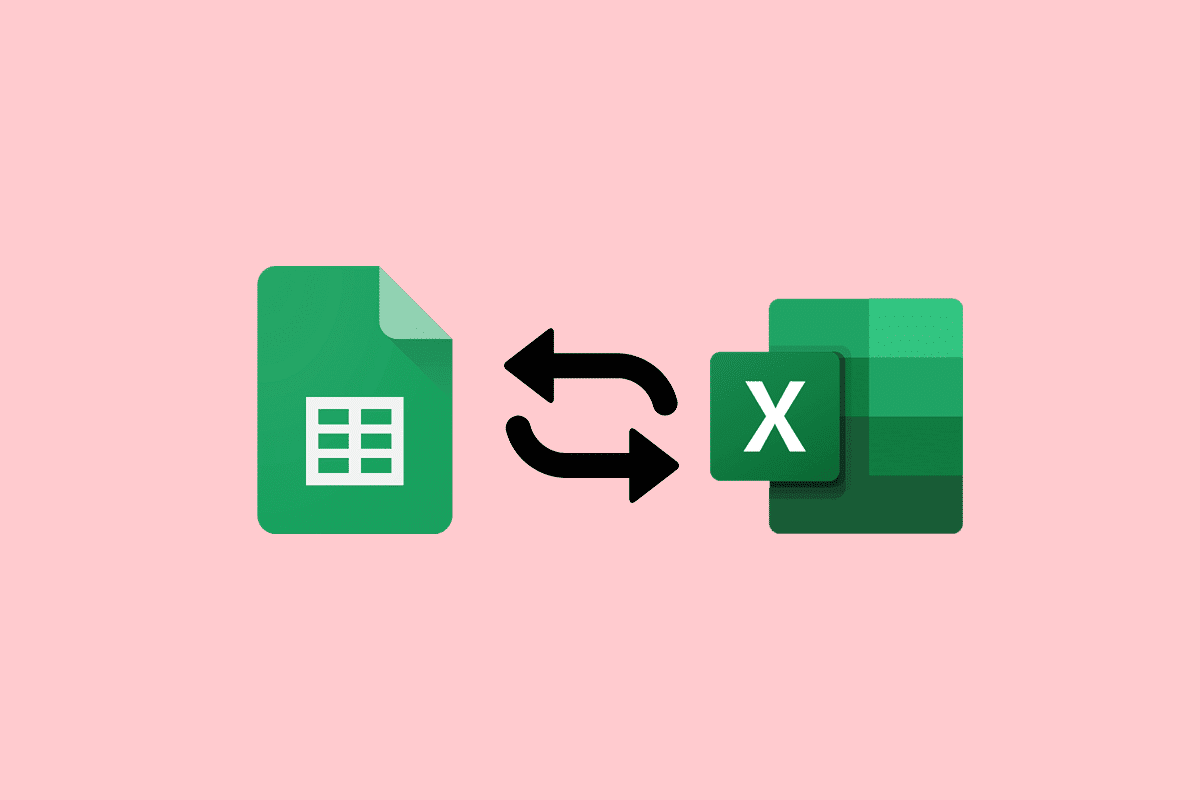## How to Convert Google Sheets to Excel Automatically

Here, you will get to know how to convert Google Sheets to Excel automatically in detail.

Is there a fast way to convert Google Sheets to Excel automatically? Yes, there is:

2. Click on File

4. Here, choose Microsoft Excel (.xlsx).

### Can you Sync Google Sheets to Excel?

Yes, you can integrate Google Sheets with Excel. You can import data from your Excel file to a new or existing Sheets file. This won’t change your Excel file, even as you change the Sheets file. Google Sheets allows users to download their spreadsheets in a format that can be opened in Microsoft Excel.

Note 1: Excel files will not automatically update if any change in the Google Sheet file is made. In this case, transform it again into the latest Excel file.

Note 2: Check the enable editing option to edit the newly created Excel file.

You can easily convert your Google Sheets to Excel manually as well as automatically. However, if you need to update the file regularly, opting for the manual method can be a bit chaotic. Manual conversion of Google Sheets to Excel is also a very tedious and time-consuming method. So, choosing automatic conversion is a wise choice in this case. You can automatically lead the file conversion from one platform to another with the help of two effective methods. If you want to know in-depth about the conversion of Google Sheets to Excel automatically, check out the methods below:

### Method 1: Through Sheet Sites

With the help of this first method, you can directly convert files from Google Sheets to Excel from its site with the help of the following steps:

1. First, click on the File tab at the top.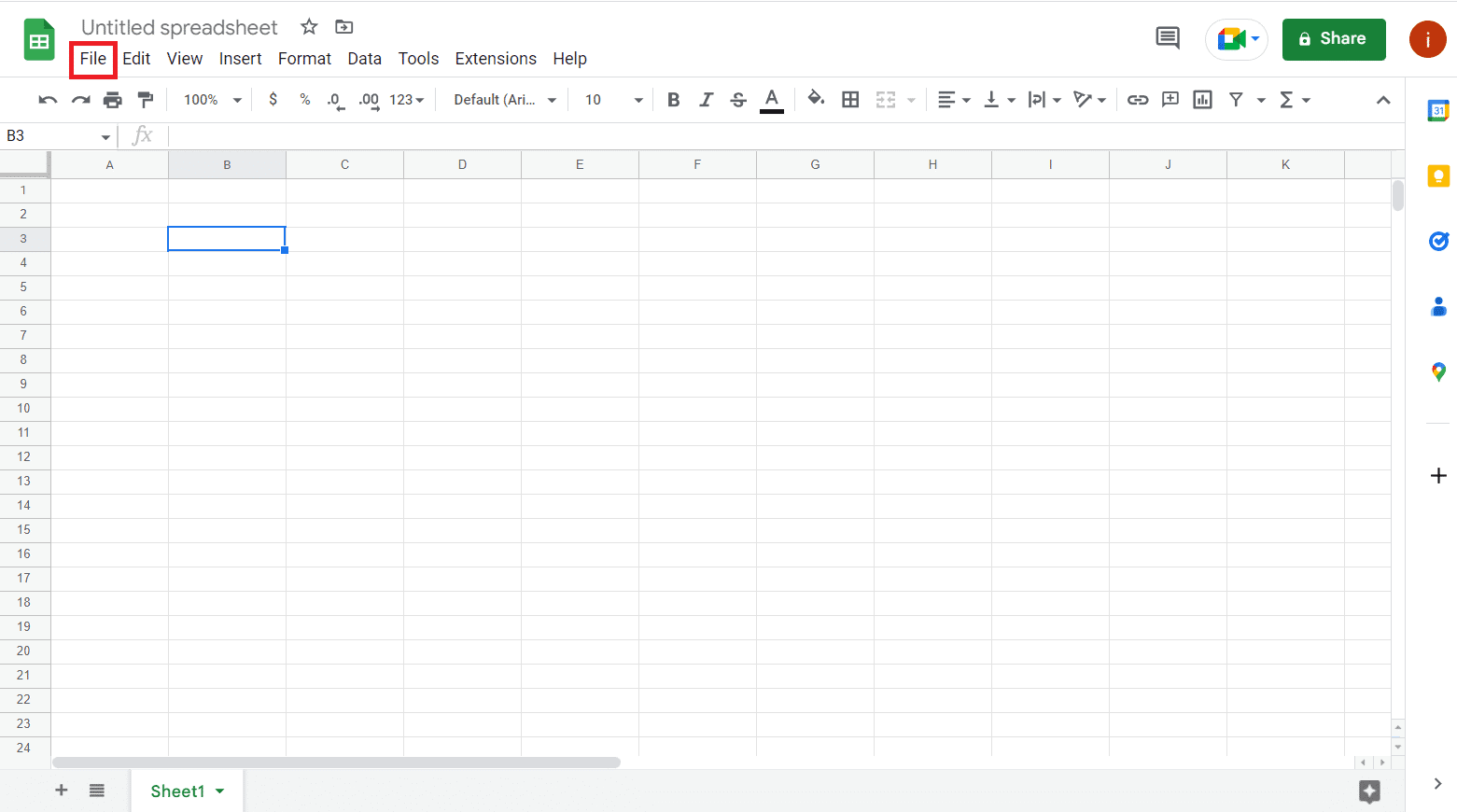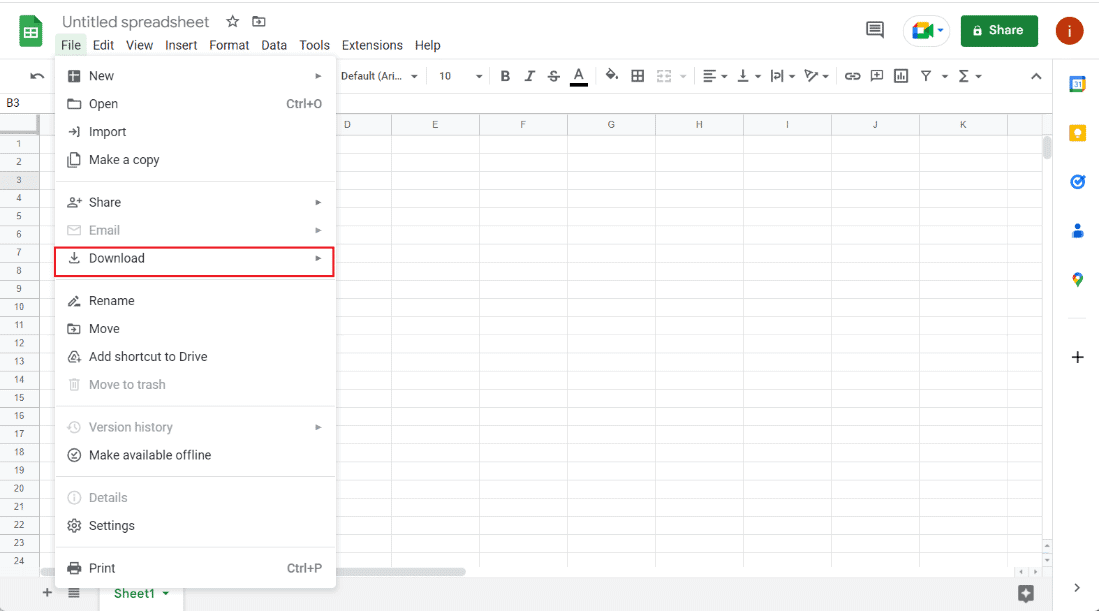3. You will see many options to download this file, click on Microsoft Excel (.xlsx) to convert it into Excel.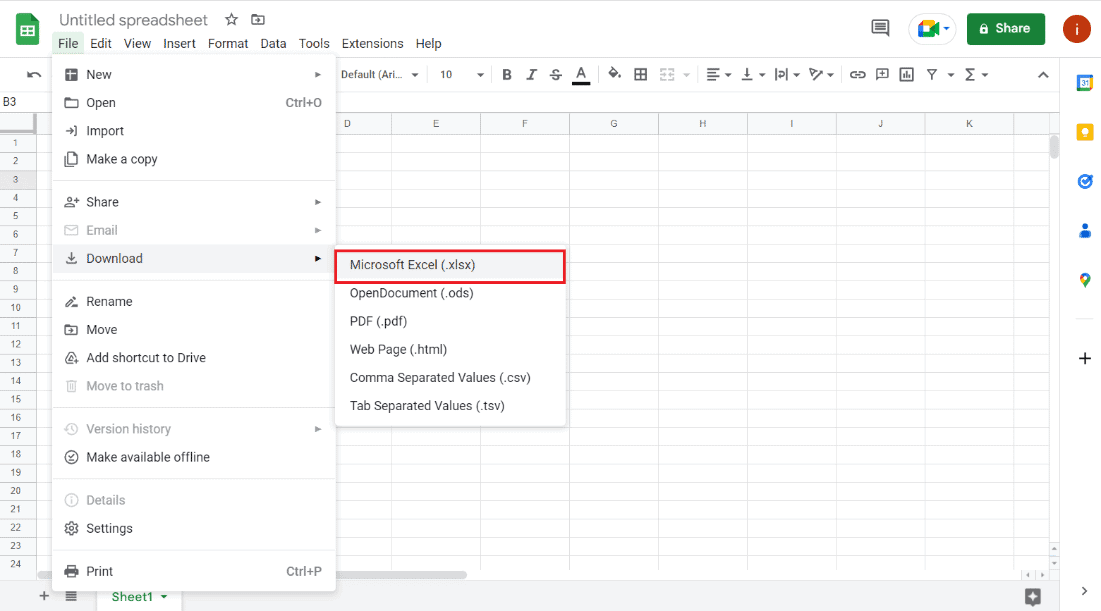Once the option has been selected, you can save the file to a preferred location and open it as an Excel file.

### Method 2: Utilize Drive Site

If you want to know how to convert Google Sheets to Excel automatically, you can also opt for the drive site to perform this action. As you might already know that the Google Sheet file is stored in the Google Drive of the file owner. Therefore, you can utilize Google Drive to download the Google Sheet file into Excel.

1. First, navigate to the Google Drive site.

2. Now, access the spreadsheet file that you want to transform into Excel.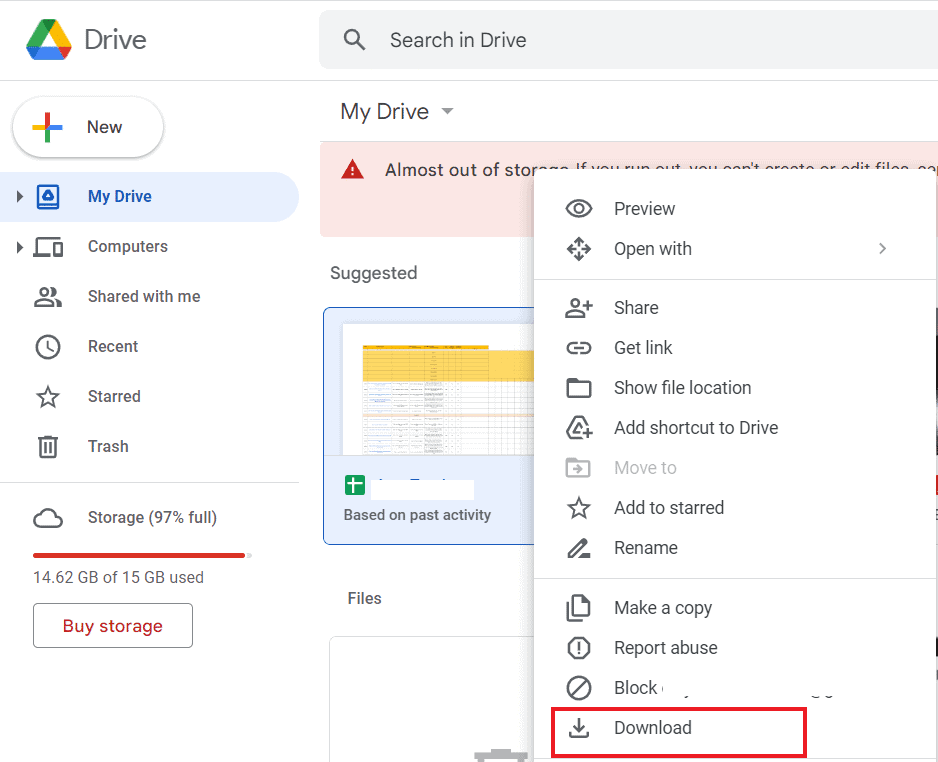Once done, your file will be saved as Microsoft Excel Worksheet.

### How to Convert Google Sheets to Excel with Formulas

You must know that there are some formulas in Google Sheets that are not compatible with Excel. Therefore, it can create problems while converting Google Sheets to Excel automatically. Therefore, let us look at some examples to convert Google Sheets to Excel with the help of the formulas below:

To start with, we will perform addition in both, Google Sheets as well as Excel. The formula changes while performing this action to convert Google Sheets to Excel. The steps below involve adding two numbers by using the add and sum functions:

1. First, select the last cell (C6) in the Google Sheets and enter the formula, =ADD(C5+C4).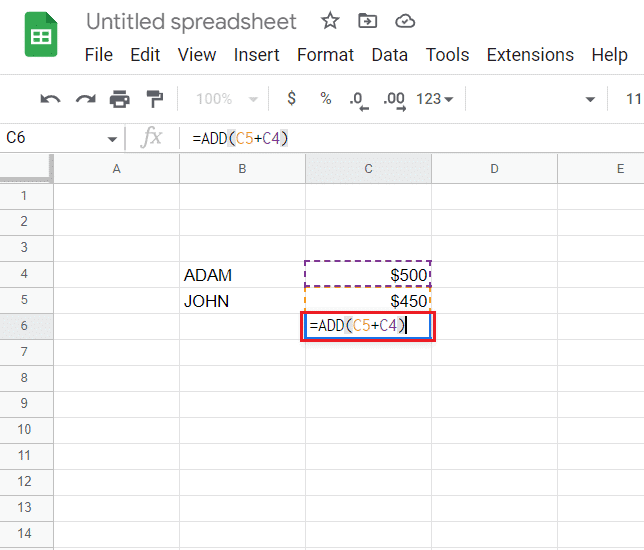2. Now, you will get the result of the addition.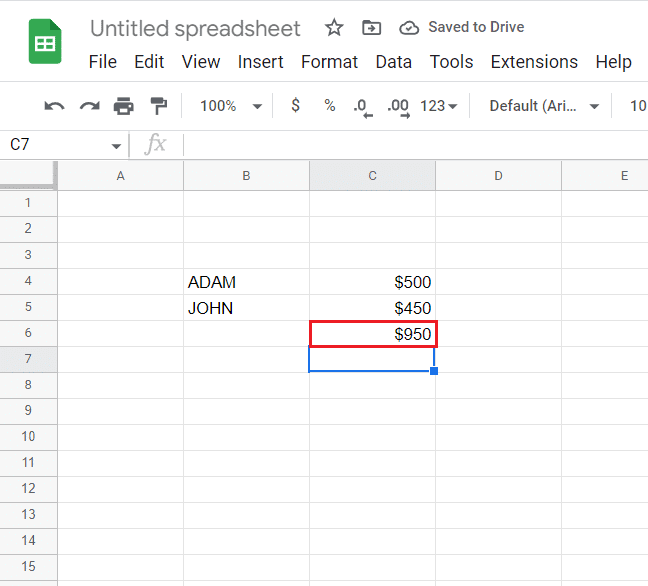3. Next, open the File tab at the top.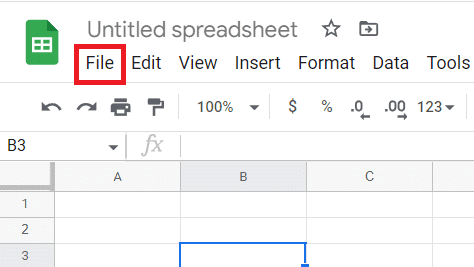5. Finally, select Microsoft Excel (.xlsx) from the next menu.6. Now, open the downloaded file to find out that the formula in Google Sheets is not compatible with Excel.

7. Then, select the C6 cell in Excel and cut the previous formula.

8. Now, enter the =SUM(C4:C5).

9. Finally, you will now get the summation of the two numbers in Excel.

#### 2. Subtract Two Values in Google Sheets and Excel

You can also convert Google Sheets to Excel with formulas with the help of subtraction, also known as the Minus function. As Excel does not have this function, on conversion, this function does not work in Excel. Therefore, to sort out the issue of the subtraction formula in Excel, check out the steps below:

1. First, select C6 cell in Google Sheets and enter the formula, =MINUS(C4,C5).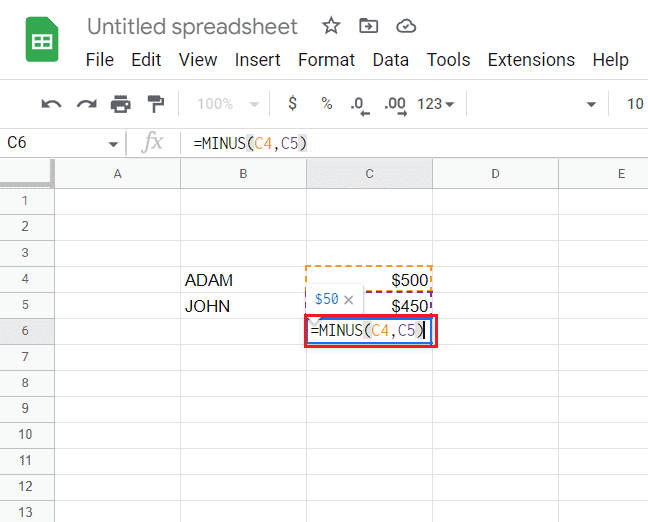2. Finally, you will get the subtraction results.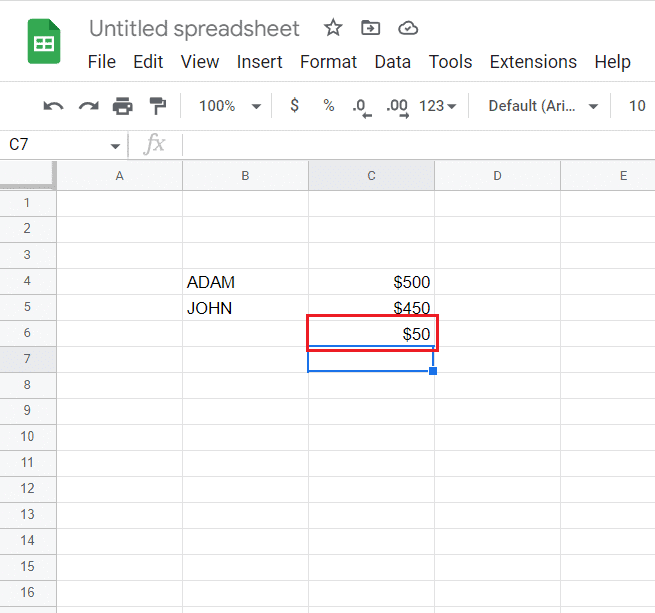4. Open the Excel file, select C6 cell and enter the formula, =C4-C5.

As a result, you will get subtraction results in Excel with a different formula.

#### 3. Divide Two Values in Google Sheets and Excel

With the help of the DIVIDE function, you can also perform division in Google Sheets and Excel. Even though Excel does not have this function, you can follow the steps below to perform the same on both platforms using different formulas:

1. First, select the C6 cell in Google Sheets and enter the formula, =DIVIDE(C4,C5).2. Once done, the division results will come.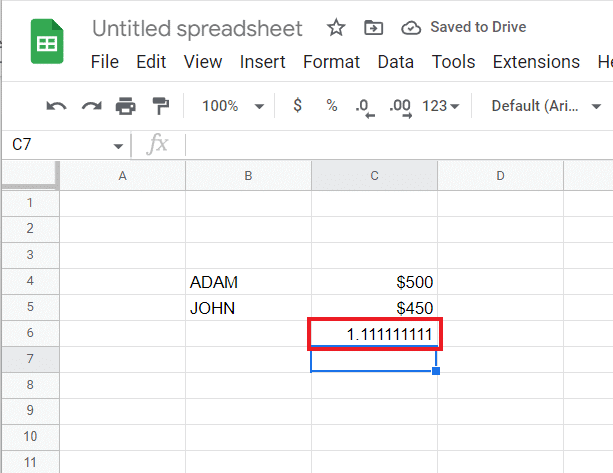3. Now, download the file to Excel with the help of steps 3 to 5 performed in the first method.

4. Next, open the Excel file, select C6, and enter, =C4/C5.

Once done, you will get the results of the division of two numbers in Excel.

#### 4. Multiply Two Values in Google Sheets and Excel

To convert Google Sheets to Excel automatically with the help of formulas, you can look into multiplication as well. It involves using the MULTIPLY function in Google Sheets which is missing in Excel. Know more about how to perform this function on both platforms with the help of the steps below:

1. First, select C6 in Google Sheets and enter the formula, =MULTIPLY(C4,C5).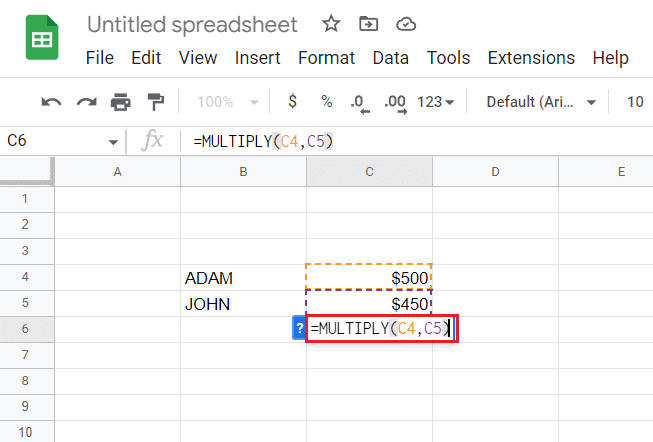2. Now, you will get the multiplication results.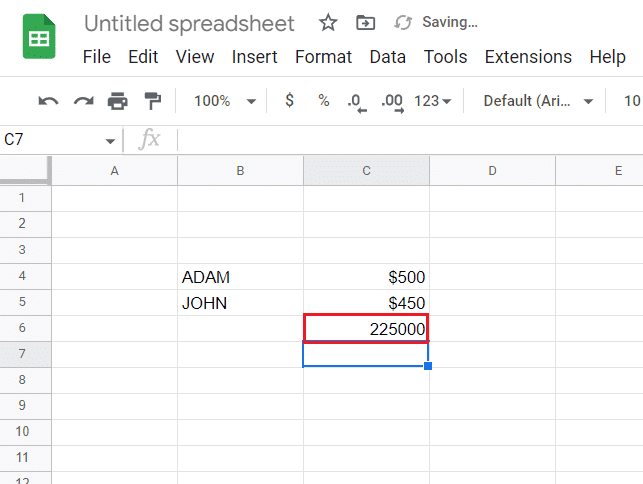3. Then, follow steps 3 to 5 performed in method 1 to download the file into Excel.

4. Now, open the Excel file, select C6 in it, and enter the formula, =C4*C5.

As a result, you will get multiplication results in Excel as well.

#### 5. Find Power to Number in Google Sheets and Excel

Finally, you can also raise a number to power in both Google Sheets and Excel with the help of the POW function in Google Sheets. On the other hand, Excel uses the POWER function to perform the same task, therefore causing incompatibility issues that are addressed in the steps below:

1. First, select C6 cell in Google Sheets and enter the formula, =POW(C4,C5).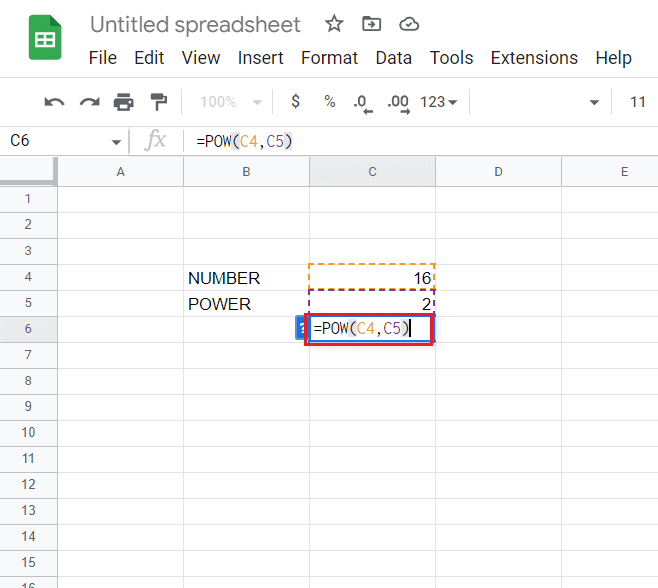2. Now, you will get the results where the C4 cell will be raised to the power of the number in the C5 cell.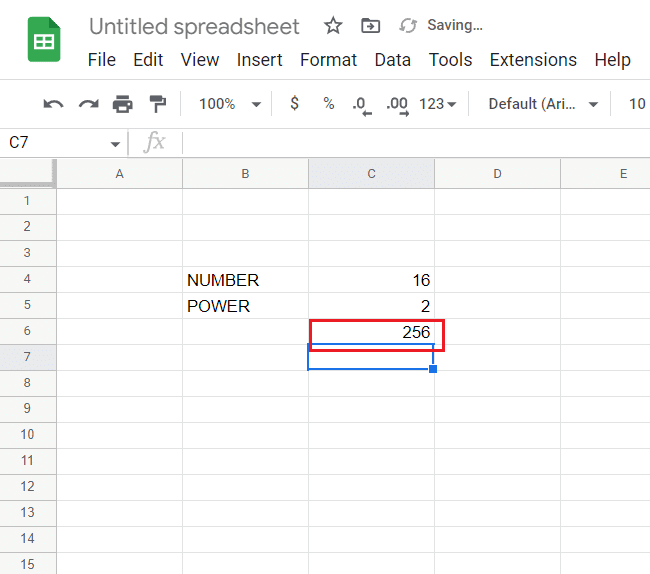4. Once done, open the Excel file, select the C6 cell, and enter =POWER(C4,C5) formula.

Finally, you will get the desired result in Excel as well.

### Why Convert Google Sheets to Excel Automatically?

While some clients and workers prefer Google Sheets to manage their data, others choose Excel to begin with. No matter which platform you choose to maintain data on, you will have to convert it to the preferred format of clients and collaborators. If you have created a Google Sheet but your collaborators or clients use Excel, you can export the file as a .xlxs.

Apart from this, the following reasons are some of the benefits of automation:

• Sync Google Sheets to Excel
• Export Google Sheets to Excel automatically
• Up-to-date Excel files.